PoundRebka Experiment Experiment Background Experiment done in 1959

• Slides: 10Pound-Rebka Experiment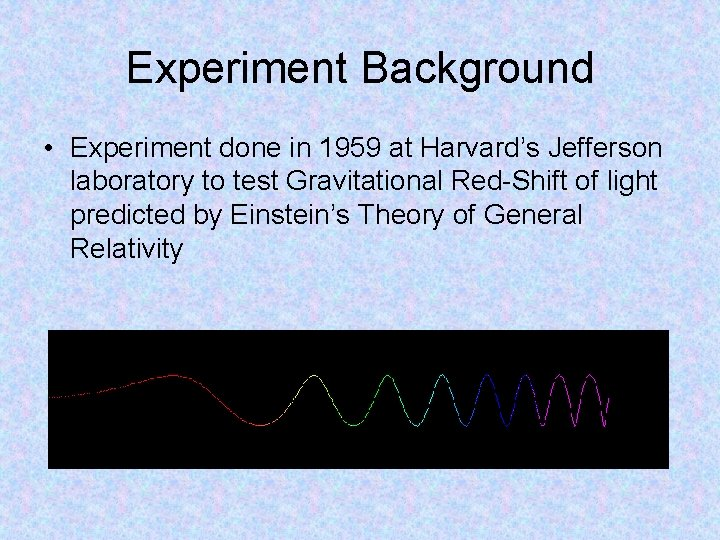Experiment Background • Experiment done in 1959 at Harvard’s Jefferson laboratory to test Gravitational Red-Shift of light predicted by Einstein’s Theory of General Relativity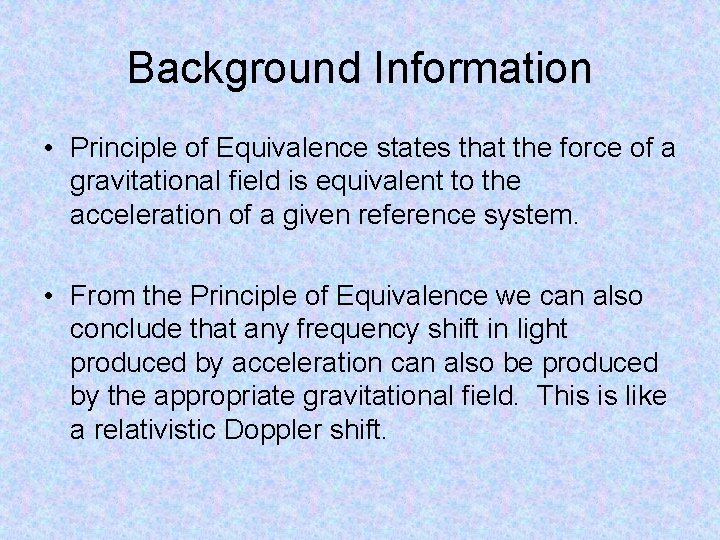Background Information • Principle of Equivalence states that the force of a gravitational field is equivalent to the acceleration of a given reference system. • From the Principle of Equivalence we can also conclude that any frequency shift in light produced by acceleration can also be produced by the appropriate gravitational field. This is like a relativistic Doppler shift.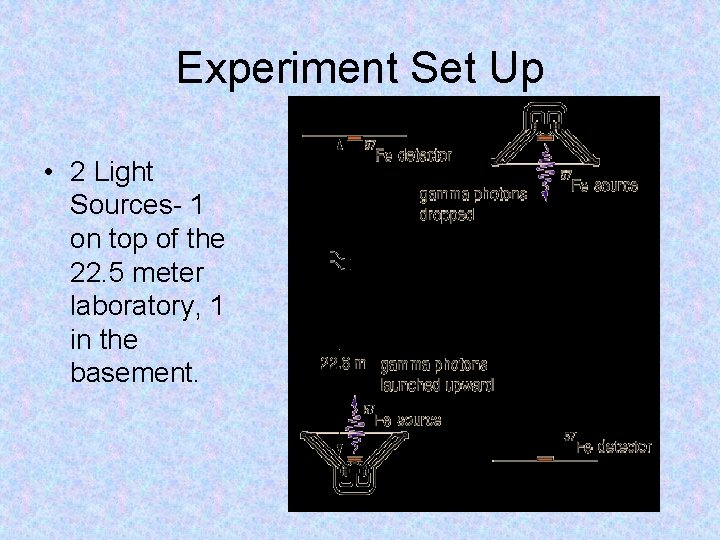Experiment Set Up • 2 Light Sources- 1 on top of the 22. 5 meter laboratory, 1 in the basement.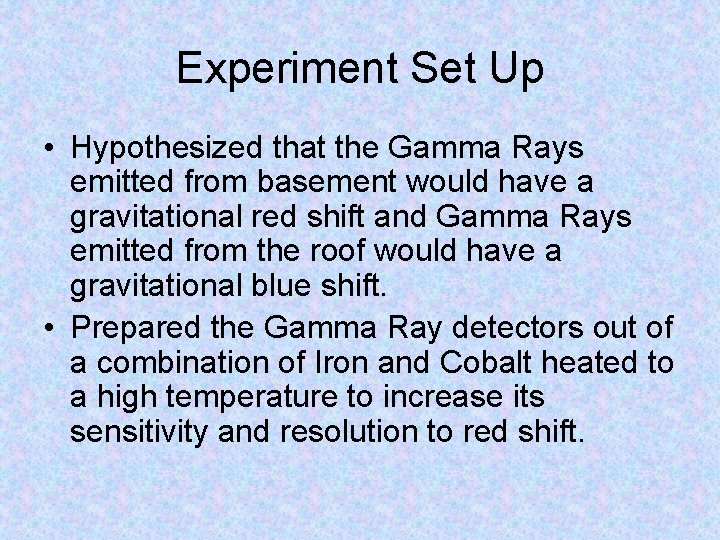Experiment Set Up • Hypothesized that the Gamma Rays emitted from basement would have a gravitational red shift and Gamma Rays emitted from the roof would have a gravitational blue shift. • Prepared the Gamma Ray detectors out of a combination of Iron and Cobalt heated to a high temperature to increase its sensitivity and resolution to red shift.Equations Used in Experiment • By using equations for gravitational potential energy and the relativistic energy expression, the gain in energy of a photon which falls a distance h is :Equations Used in Experiment • Difference in energy shifts from the 2 gamma ray emissions was predicted to be: • While it was measured as: •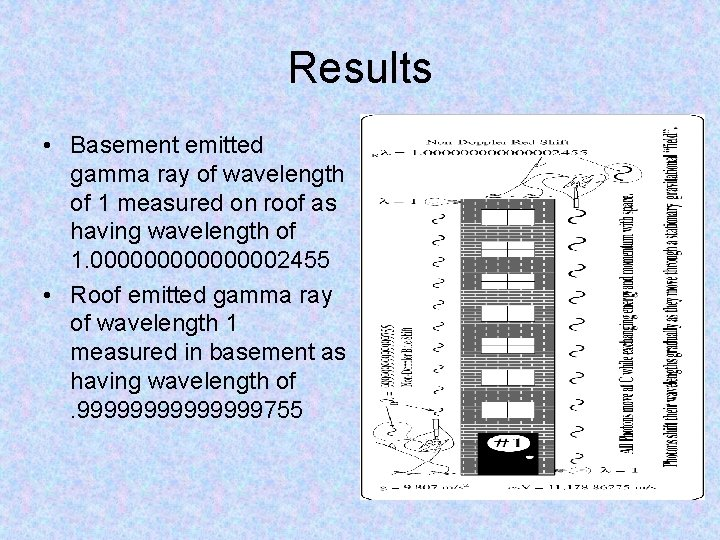Results • Basement emitted gamma ray of wavelength of 1 measured on roof as having wavelength of 1. 00000002455 • Roof emitted gamma ray of wavelength 1 measured in basement as having wavelength of. 9999999755Implications • The measured energy difference and gravitational shift was within the error margin of the predicted value by General Relativity • Experiment claimed as Proof of General RelativityBibliography • “Harvard Gravitational Red Shift Experiment. ” http: //www. circlontheory. com/HTML/pound. Rebka. html • “Equivalence Principle. ” http: //en. wikipedia. org/wiki/Equivalence_pr inciple • “Gravitational Red Shift. ” http: //hyperphysics. phyastr. gsu. edu/hbase/relativ/gratim. html